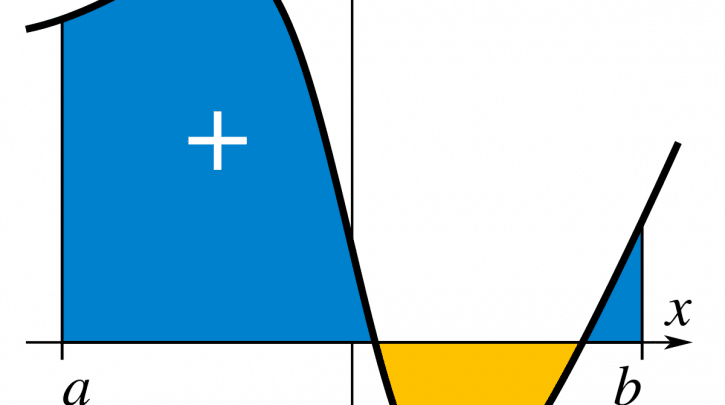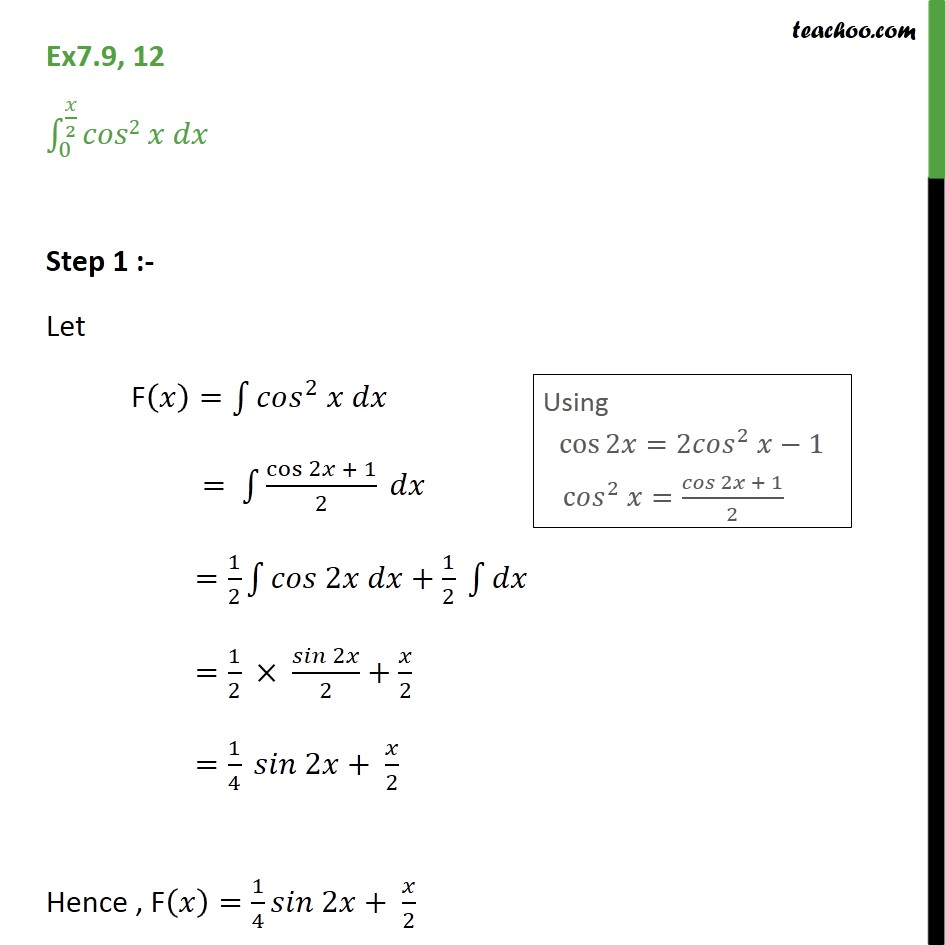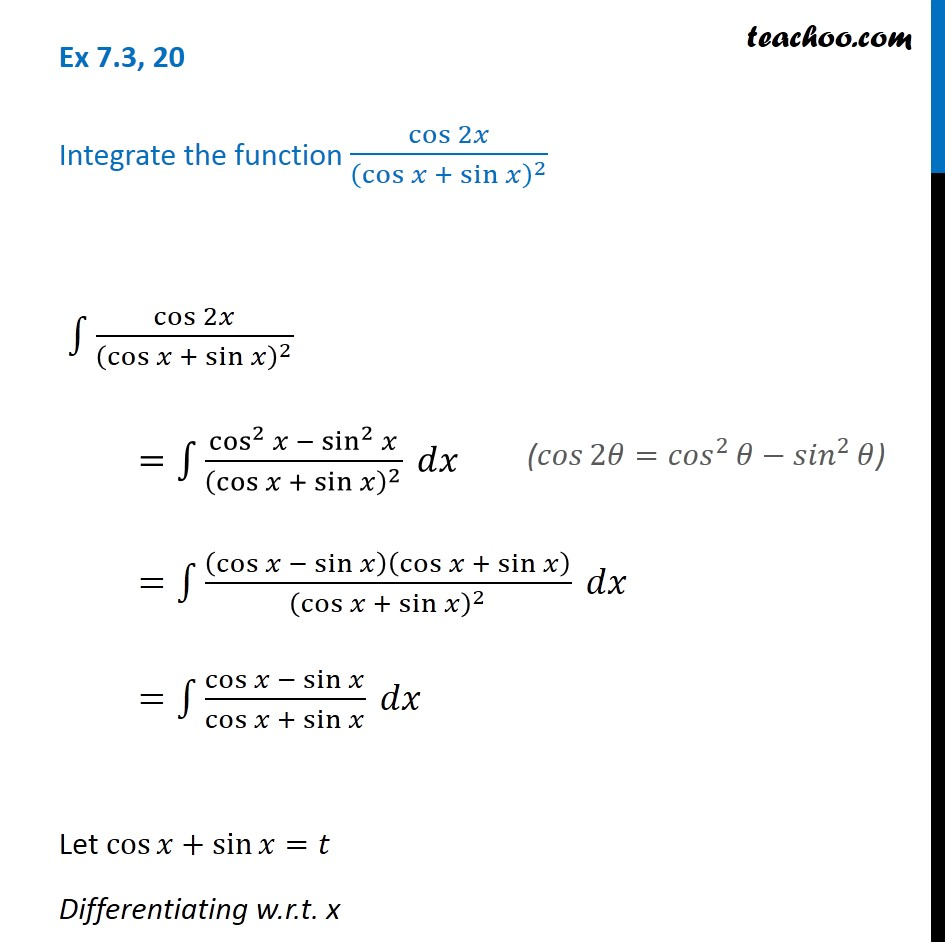# The Integral of cos2x: Definition, equation, formula and more

## What is integral

Normally the integral means an area. It is true in some cases, the number that we get when we evaluate an integral represents an area. The Riemann sum represents the total area of a number of rectangles. If we increase the number of rectangles, they will cover the region between the graphs. However, they will cover the horizontal axis too. In this case, the area of the region is equal to the limit of the Riemann sum. Now that limit is precisely the integral of the function over the interval. So, in this case, the interval is an area.But in general, it is different. Problems arise when the function becomes negative at certain points. However, at points where the function is negative, the corresponding terms in the Riemann sum will also be negative. However, the Riemann sum represents the total area of the rectangles above the axis minus the total area of the rectangles below the axis. The graph, the horizontal axis, and the lines x= a, and x= b enclose a region. Now, the integral represents the total area B of the part above the horizontal axis minus the area A of the part below the axis. Next It is the signed area of the region. So, the actual meaning of integral is the signed area of the region enclosed by the graph.

### Integral of cos^2x

Cosine squared x is the same as one-half the quantity one plus cosine 2x. Now we are reducing the square. So we will pull that one half out front. Then we can factor constants out of integrals, and this will be the antiderivative of one plus cosine of 2x. Now, this is something that we can actually anti derive. We have a one-half; the antiderivative of one dx is simply x. Now, the antiderivative of plus a cosine is plus a sine. However, If we were deriving, we would have to multiply by two by the chain rule. Next, we need to divide by 2. However, that only works when it’s a constant time x on the inside. An indefinite integral deserves plus c.

∫ cos²x dx = 1/2 ∫ 2cos²x dx

= 1/2 ∫ (1+cos2x) dx

=1/2 { ∫ dx + ∫ cos2x dx } =1/2 (x + sin2x/2) + C### Integral of cos^2x sin^2x

• So we need to convert the sine square x to 1 minus cosine squared x. Next, cosine squared times 1 is cosine square, and this x does cosine to the fourth power x. However, we cannot use u-substitution because if we want to let u equal to sine x, the derivative sine x is cosine x. Now, we have cosine to the second power x. Now rewrite the integral as the integral of sine x times cosine x. Then take both of that to the second power. Because when we have sine x cosine x, it’s nothing that we can recognize. Now we have to divide both sides by 2. So this is the integral of ½ times sine of 2x and squares them both. Now we have a ¼ to the front, and then we have to integrate. Next, the function part is sine, and we have to square it.
• After that, put 2x states inside the brackets. Now, 2x is the constant multiple with x and let u equal to 2x. Now we are using the power reduction formula for sine square. We can change sine squared theta to ½ times one minus cosine of the angle in shires two times theta. Next, we can bring ½ to the front, and ¼ times one over two is one over 8. Then we can integrate one minus cosine two times 2x which is for x. Then we can bring ⅛ to the front and open the parentheses; we get x and integrate the cosine, we get a positive sign. However, input states the same because the input was 4x. So finally, we have ⅛ x minus 1/32x sine 4x plus c.

sin^2xcos^2xdx=1/4∫(4sin^2x cos^2x)dx

= 1/4∫sin^2(2x)dx

= 1/4∫1−cos4x/2dx

= x/8−18∫cos4xdx

= x/8−18×sin4x/4+c

= x/8−sin4x/32+c

image

#### Integral of cos^2x from 0 to pi

Cosine square is the same as ½ times one plus cosine of 2 thetas, and this is going to be much easier to integrate. Now the ½ will be inside, but then the number we can bring that outside one-half integral 0 is over 2. Next, it will be just one plus cosine two theta d theta, and then we can integrate this. However, the ½ sits on the outside and opens the parentheses. Then, the integral of 1 theta in the theta world plus by the sine and inside the states, it is two thetas. Now we have to divide it by two. At last, the answer is π/4.### Integral of cos2x/(cosx+sinx)^2

We integrate the function, which is cos2x divided by cos x plus sine x, to the power two dx. Now we are breaking the numeral using cos2x. However, we know that cos2x equals cos square x minus sine square x. Next, we are applying this formula in the numeral, and this becomes cos square x minus sine squared x divided by cos x plus sine x and total to the power two into dx. Next step, we are going to split the numerator with the formula of (a squared minus b square). Now that will become (cos x minus sine x) into (cos x plus sine x) divided by cos x plus sine x and total to the power two into dx. Next, after canceling the cos x plus sine x, it will be cos x minus sine x divided by cos x plus sine x into dx.

Let, cosx + sinx = t

(-sinx + cosx) dx= dt

After replacing it with t, the question will be dt upon t. Now the integral of the function is the log mod of x plus c. Next, we integrate this function, and it will be a log mod of t plus c. Now the result is the log mod of cos x plus sine x plus c.

I=∫cos2x/(sinx+cosx)2 dx

I=∫cos2x/(sinx+cosx)2dx

I=∫cos2x−sin2x/(sinx+cosx)2dx

I=∫(cosx+sinx)(cosx−sinx)/(sinx+cosx)2dx

I=∫cosx−sinx/sinx+cosx dx

Let sinx+cosx=t Then, (cosx−sinx)dx=dt

Then,

I=∫dt/t

I=log|t|+c

I=log|sinx+cosx|+c#### Integral of dx/cos(x+a)cos(x+b)

• We integrate the function, which is dx over cos of x plus a and cos of x plus b. Now we need to multiply numerator and denominator with the sine of a minus b. After this, it will be the sine of a minus b divided by cos of (x+a) cos of (x+b) into dx. Now we will further manipulate this format and get sine of (a-b). In the numerator, we will get sine of (a+x-b-x). And in the denominator, we will get the cos of (x+a) into cos of (x+b) into dx. In the next step, the sine of (a+b) will be the same. But the numerator and denominator will be the sine of (x+a-x-b) and cos of (x+a) into cos of (x+b) into dx. However, the sin of (a-b) equals sinA cosA minus cosA sine B.
• After applying this formula, we will get the sine of (x+a) into cos of (x+b) minus cos of (x+a) into sine of (x-b) divided by cos of (x+a) into cos of (x+b) into dx. Next, after some calculation, it will be the sine of (a-b), and now it’s integral of tan of (x+a) into dx minus 1/sine of (a-b). Again integral of tan of (x+b) into dx. Next, after the integral of tan and applying log, the final result is one upon sine of (a-b) and log total mod of cos(x+b) upon cos(x+a) plus c.

∫ 1/cos(x+a) cos(x+b) dx

= 1/sin(a-b) ∫ tan(x+a) – tan(x+a) dx

= 1/sin(a-b) [-log|cos(x+a)| + c1 -(-log|cos(x+b)| + c2)

= 1/sin(a-b) [-log|cos(x+a)| + log|cos(x+b)|+ c1 + c2]

= 1/sin(a-b) [-log|cos(x+a)| + log|cos(x+b)|] + 1/sin(a+b) (c1+c2)

= 1/sin(a-b) [-log|cos(x+a)| + log|cos(x+b)|] + c

= 1/sin(a-b) log|cos(x+b)/cos(x+a)| + c

#### Some frequently asked questions

##### What is the integration of LOG X?

X log x−x+c is the integration of log x.

##### What is the Antiderivative of COSX?

SinX is the antiderivative of cosx.

##### What is the integration of log 2?

∫udv=uv−∫vdu ∫ u d v = u v – ∫ v d u is the integration of log 2, where u =log2(x) u = log 2 ( x ) and dv=1 d v = 1.

##### What is the integral of cos 2x sin 2x DX?

∫ (Sin 2x Cos 2x) = (Sin 2x) 2 / 4 + C is the integral of cos 2x sin 2x dx.

##### What is the formula of sin2x?

2sinxcosx is the formula of sin2x.

##### What is the derivative of cos 2x?

-2sin(2x) is the derivative of cos 2x.

##### What is the tan2x formula?

2tan x / (1−tan2x) tan 2x is the formula of tan2x.